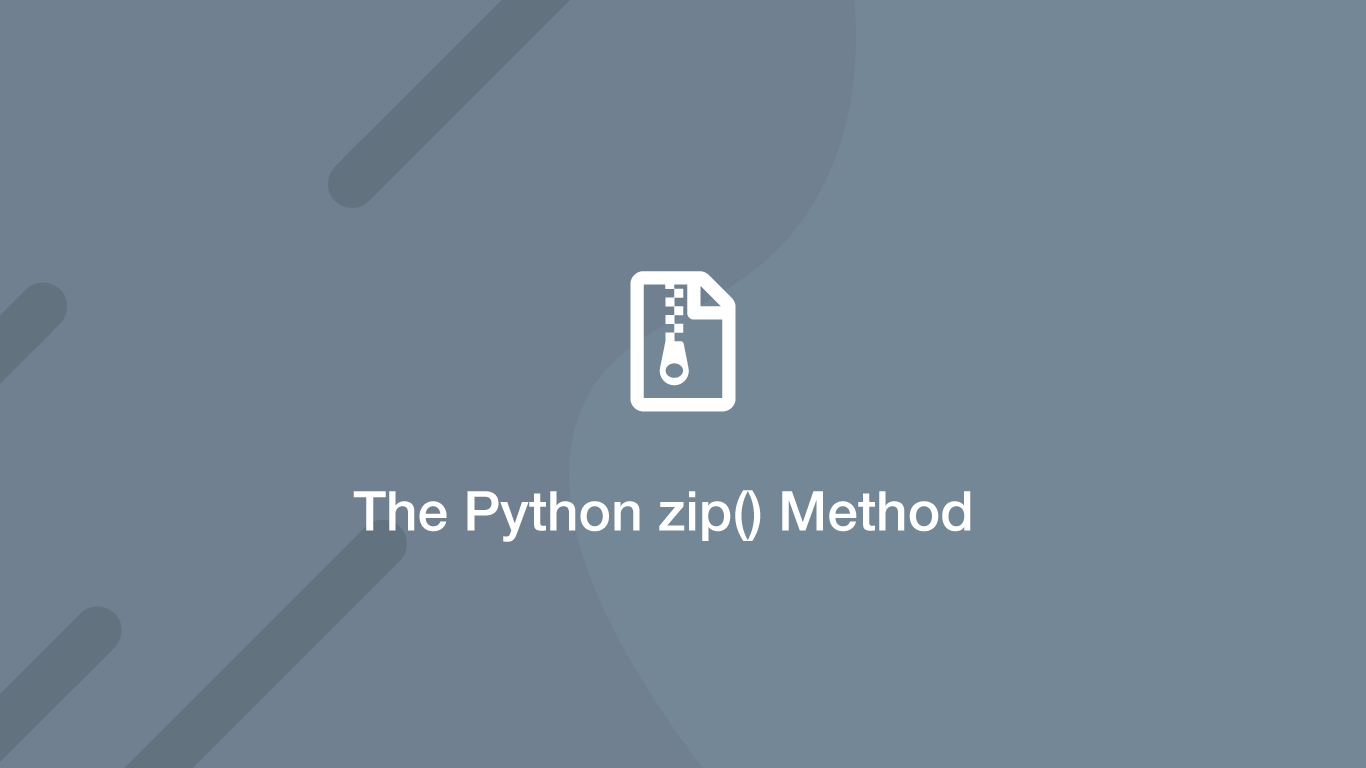# The Python zip() MethodThe Python `zip()` method is used to combine iterables into an object of tuples. What makes this method powerful is that any type of iterables can be combined together and can be unzipped further in the program.

In this tutorial, we will learn how to use the Python `zip()` method using examples or zipping iterables and unzipping them.

## The Python zip() Syntax

Python `zip()` method take zero or multiple iterables (list, tuple, dictionary) in a comma-separated list.

``````zip(iterable_1, iterable_2)
``````

## Creating an Empty Zip Object

The most basic way to use `zip()` is to create an empty `zip` object. This is done by calling `zip()` with no arguments.

``````result = zip()

print(type(result))
``````
``````<class 'zip'>
``````

## Zipping a Single Iterable

First, let's try zipping a single iterable and see what the result is. We will have to convert the `zip` object into a list before it can be printed.

``````items = ['a', 'b', 'c']
result = zip(items)
result = list(result)
print(result)
``````
``````[('a',), ('b',), ('c',)]
``````

## Zipping Iterables Together

Now let's try zipping multiple iterables together and see what output we get.

``````items = ['a', 'b', 'c']
words = ('one', 'two', 'three')
nums = [1, 2, 3]

result = zip(items, words, nums)
result = list(result)
print(result)
``````
``````[('a', 'one', 1), ('b', 'two', 2), ('c', 'three', 3)]
``````

In the example above we can see that `zip()` combines elements from the same index and puts them in a tuple in the order that each iterable was supplied to `zip()`.

## Zipping Iterables with Different Lengths

If iterables with different lengths are supplied, `zip()` will only create tuples up to the length of the shortest iterable. Let's try this out with an example.

``````items = ['a', 'b', 'c']
words = ('one', 'two')
nums = [1, 2, 3]

result = zip(items, words, nums)
result = list(result)
print(result)
``````
``````[('a', 'one', 1), ('b', 'two', 2)]
``````

## Unzipping Zipped Values

To unzip values supply a comma-separated list of variables to assign the unzipped tuples to and inside `zip()` supply an asterisk before the `zip` object.

``````items = ['a', 'b', 'c']
words = ('one', 'two', 'three')
nums = [1, 2, 3]

result = zip(items, words, nums)
result = list(result)
print(result)

a,b,c = zip(*result)

print(a)
print(b)
print(c)
``````
``````[('a', 'one', 1), ('b', 'two', 2), ('c', 'three', 3)]
('a', 'b', 'c')
('one', 'two', 'three')
(1, 2, 3)
``````

## Conclusion

You now know how to use the `zip()` method in Python to join multiple iterables into a `zip` object of tuples and then unzip them.

#### Related Tutorials### Unzip Files in Python

June 30, 2021### The Python zip() Function

October 04, 2020### How to Unzip Files in Linux

June 03, 2020### How to Open a ZIP file in Python

April 29, 2021June 22, 2021